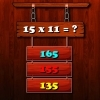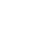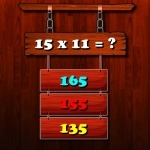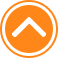×

PlayMultiplication Math Challenge81%Device: 💻 Pc   📱 Mobile
Rating: 81%
🎲Multiplication Math Challenge

Multiplication Math Challenge is an online game that you can play for free. Multiplication Math Challenge is a game of multiplication. You have 10 seconds for each questions. Your brain needs to think quickly to find the right answer and get points. Have a good time. Here you can play Multiplication Math Challenge game and observe its walkthrough at no cost in friv1000.com. Multiplication Math Challenge is an wonderful game from our list of Friv 1000 games.

🎮 Instructions

Use Left Mouse button to Play

📂 Categories

thinking, arcade, puzzle, math, mathka, numbers, school, skill, skills, mathematical, mathematics, multiplication, mathematic, brain, ..

👌 Recommended
📌 Tags